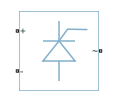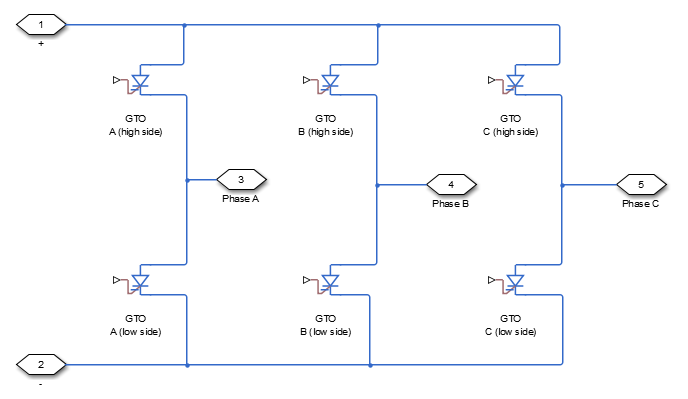# Average-Value Inverter (Three-Phase)

Average-value DC Voltage to three-phase AC voltage converter with fixed power loss

• Library:
• Simscape / Electrical / Semiconductors & Converters / Converters

•## Description

The Average-Value Inverter (Three-Phase) block models an average-value, full-wave inverter. It converts DC voltage to three-phase AC voltages and converts three-phase AC power demand to DC power demand. The corresponding DC power demand is equal to the sum of the fixed power loss and the AC power demand.

You can use the Average-Value Inverter (Three-Phase) block only as a full-wave inverter. It behaves as a DC-voltage-controlled AC voltage source. The ratio you specify determines the ratio between the DC voltage and the AC voltage.

The figure shows the equivalent circuit for the inverter as a full-wave inverter. The Average-Value Inverter (Three-Phase) block does not yield the harmonics that are typically associated with the detailed representation, however, because it performs an average-value power conversion.### Electrical Defining Equations

The voltages are defined by

`${v}_{DC}={v}_{p}-{v}_{n},$`

`${v}_{ref}=\frac{{v}_{p}+{v}_{n}}{2},$`

`${v}_{RMS}={v}_{ratio}{v}_{DC},$`

`${V}_{0}=\frac{\sqrt{2}}{\sqrt{3}}{V}_{RMS},$`

`${v}_{a}={V}_{0}\mathrm{sin}\left(2\pi ft+\phi \right)+{v}_{ref},$`

`${v}_{b}={V}_{0}\mathrm{sin}\left(2\pi ft+\phi -{120}^{\circ }\right)+{v}_{ref},$`

and

`${v}_{c}={V}_{0}\mathrm{sin}\left(2\pi ft+\phi +{120}^{\circ }\right)+{v}_{ref},$`

where:

• vp and vn are the voltages at the positive and negative terminals of the inverter.

• vDC is the voltage difference between the positive and negative terminals of the inverter.

• vref is the DC offset.

• Vratio is the ratio of rated AC voltage to rated DC voltage for the inverter. See the Ratio of rated AC voltage to rated DC voltage parameter in Parameters for the Vratio values for common inverter control modes.

• VRMS is the RMS AC line-line voltage.

• V0 is the peak phase voltage.

• f is the frequency.

• t is the time.

• φ is the phase shift.

• va, vb, vc are the respective AC phase voltages.

The power, resistance, and currents are defined by

`${P}_{AC}=-{v}_{a}{i}_{a}-{v}_{b}{i}_{b}-{v}_{c}{i}_{c},$`

`${R}_{DC}=\frac{{v}_{DC}^{2}}{{P}_{AC}+{P}_{fixed}},$`

and

`$i=\frac{{v}_{DC}}{{R}_{DC}},$`

where:

• ia, ib, and ic are the respective AC phase currents flowing into the inverter.

• PAC is the power output on the AC side. PAC has a minimum limit of `0` W.

• Pfixed is the fixed power loss that you specify on the block.

• RDC is the resistance on the DC side.

• i is the current flowing from the positive to the negative terminals of the inverter.

The inverter starts to create an AC voltage, that is turns on, when the DC supply voltage is above the value that you specify for DC voltage for turn on parameter. It stops inverting, that is turns off, when the DC supply voltage falls below the value that you specify for DC voltage for turn off parameter. When the inverter turns off, the block sets the output AC current to zero.

## Ports

### Conserving

expand all

Electrical conserving port associated with the positive terminal

Electrical conserving port associated with the negative terminal

#### Dependencies

To enable this port, set Electrical connection to ```Composite three-phase ports```.

Electrical conserving port associated with a-phase.

#### Dependencies

To enable this port, set Electrical connection to ```Expanded three-phase ports```.

Electrical conserving port associated with b-phase.

#### Dependencies

To enable this port, set Electrical connection to ```Expanded three-phase ports```.

Electrical conserving port associated with c-phase.

#### Dependencies

To enable this port, set Electrical connection to ```Expanded three-phase ports```.

## Parameters

expand all

Whether to have composite or expanded three-phase ports.

AC frequency, specified in Hz (where Hz is defined as $1/s$). For example, kHz and MHz are valid units, but rad/s is not.

Phase shift in angular units.

The table shows ratios for common three-phase two-level inverter control modes. The default value is $\sqrt{6}/\pi$.

For 180° and 120° conduction modes, the listed voltages are the fundamental RMS values of line-line voltages. For other methods, the listed voltages are the maximum fundamental RMS values of line-line voltages.

You can control the output voltage of the inverter according to specific requirements. DPWM includes 30° DPWM, 60° DPWM, and 120° DPWM. For details, see references  and .

Control Method

V RMS (line-line) Ratio of V RMS (line-line) to v DC
180° conduction mode  $\frac{\sqrt{6}}{\pi }{V}_{DC}$

0.7797

120° conduction mode  $\frac{3}{\sqrt{2}\pi }{V}_{DC}$

0.6752

Hysteresis current control 

$\left(\frac{\sqrt{3}}{\sqrt{2}}\right)\left(\frac{2{V}_{DC}}{\pi }\right)$

0.7797

Sinusoidal PWM (SPMW) 

$\left(\frac{\sqrt{3}}{\sqrt{2}}\right)\left(\frac{{V}_{DC}}{2}\right)$

0.6124

Space vector modulation (SVM) 

$\left(\frac{\sqrt{3}}{\sqrt{2}}\right)\left(\frac{{V}_{DC}}{\sqrt{3}}\right)$

0.7071

Discontinuous PWM (DPWM) , 

$\left(\frac{\sqrt{3}}{\sqrt{2}}\right)\left(\frac{{V}_{DC}}{\sqrt{3}}\right)$

0.7071

Convert to the original AC voltage of the average-value rectifier

$\left(\frac{\pi }{\sqrt{2}}\right)\left(\frac{{V}_{DC}}{3}\right)$

0.7405

Minimum power drawn on the DC side. The default value is `1e3`.

When the DC supply voltage rises above this value, the inverter produces an AC output voltage.

When the DC supply voltage falls below this value, the inverter turns off and the block sets the output AC currents to zero.

 Rashid, M. H. Pulse-Width-Modulation Inverters. Upper Saddle River, NJ: Prentice-Hall, 2004, pp. 237–248.

 Krause, P. C., O. Wasynczuk, and S. D. Sudhoff. Analysis of Electric Machinery and Drive Systems. Piscataway, NJ: IEEE Press, 2002.

 Chung, D. W., J. S. Kim, and S. K. Kul. “Unified voltage modulation technique for real-time three-phase power conversion.” IEEE Transactions on Industry Applications. Vol. 34, no. 2, 1998, pp. 374–380.

 Hava, A. M., R. J. Kerkman, and T. A. Lipo. “Simple analytical and graphical methods for carrier-based PWM-VSI drives.” IEEE Transactions on Power Electronics. Vol. 14, 1999, no. 1, pp. 49–61.